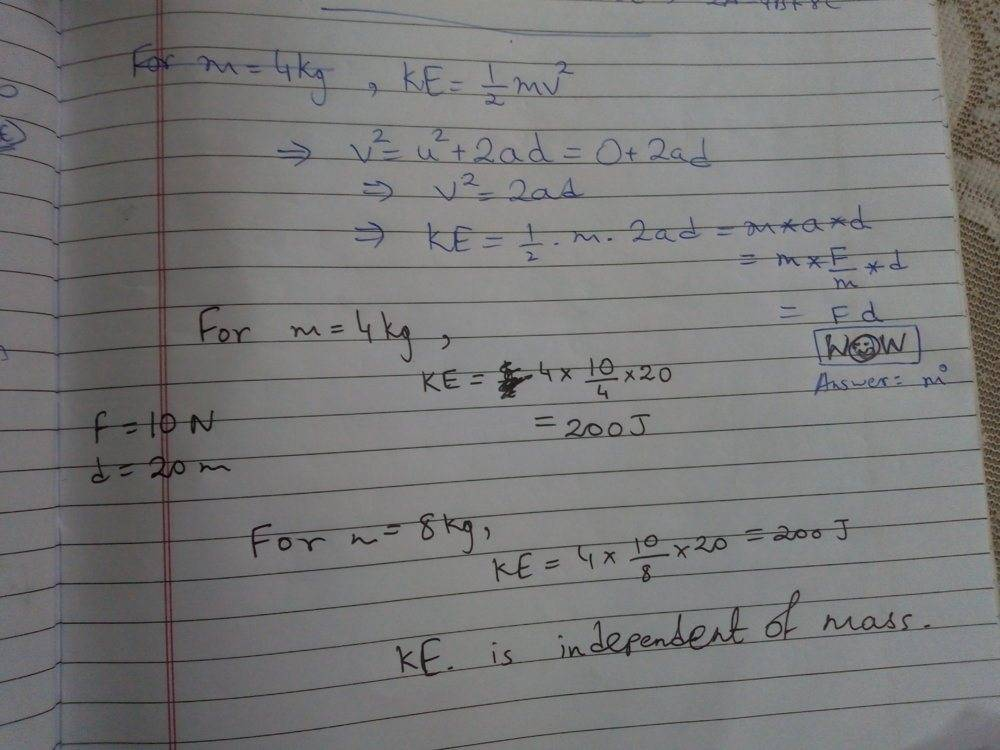# Work, Energy and Power

• Rongeet Banerjee
Rongeet Banerjee
Homework Statement
The kinetic energy acquired by a mass m in travelling distance d, starting from rest,under the action of a constant force is directly proportional to
1.m
2.m to the power 0
3.m to the power 0.5
4.m to the power -0.5
Relevant Equations
1/2m(v² -u²)=F*d
F=m*a
v²-u²=2a*d
Initial velocity u=0
Let Final velocity be v
Then, KE acquired by the object=1/2mv²
So the answer must be option1 i.e. m¹
But my textbook says the answer is- m to the power zero.Please explain this discrepancy.

Homework Helper
Gold Member
2022 Award
Homework Statement:: The kinetic energy acquired by a mass m in traveling distance d, starting from rest,under the action of a constant force is directly proportional to
1.m
2.m to the power 0
3.m to the power 0.5
4.m to the power -0.5
Relevant Equations:: 1/2m(v² -u²)=F*d
F=m*a
v²-u²=2a*d

Initial velocity u=0
Let Final velocity be v
Then, KE acquired by the object=1/2mv²
So the answer must be option1 i.e. m¹
But my textbook says the answer is- m to the power zero.Please explain this discrepancy.
Your answer says that the greater the mass the greater the kinetic energy. Is this what you mean?

How do you interpret the book's answer?

•Rongeet Banerjee
Homework Helper
Gold Member
Homework Statement:: The kinetic energy acquired by a mass m in traveling distance d, starting from rest,under the action of a constant force is directly proportional to
1.m
2.m to the power 0
3.m to the power 0.5
4.m to the power -0.5
Relevant Equations:: 1/2m(v² -u²)=F*d
F=m*a
v²-u²=2a*d

Initial velocity u=0
Let Final velocity be v
Then, KE acquired by the object=1/2mv²
So the answer must be option1 i.e. m¹
But my textbook says the answer is- m to the power zero.Please explain this discrepancy.
You must look at how the KE changes if the mass changes, assuming that the force and distances remain the same. How does the value of the KE change if you change the mass but keep F and d the same?

If it is not clear, try with some number, say F=10 N and d=20 meters. Assume m= 4 kg. What is the kinetic energy acquired? Now if you have a mass of 8kg, what will be the change of KE this time?

•Rongeet Banerjee
Part of the issue seems to be in determining which other quantities are dependent on mass. For a fixed velocity, KE is indeed proportional to ##m##. But in this problem, the acceleration and by extension the velocity after any given distance is also related to the mass.

You want to find a relation in which everything apart from KE (and ##m##) is independent of ##m##.

•Rongeet Banerjee
Rongeet Banerjee
Thank You verrrrrrrrry much nrqed 😁.
That was a fantastic explanation.Miraculous .I Love Physics...•nrqed
Rongeet Banerjee
Your answer says that the greater the mass the greater the kinetic energy. Is this what you mean?

How do you interpret the book's answer?
Now I get it.Thank You for your help.

•PeroK
Rongeet Banerjee
Part of the issue seems to be in determining which other quantities are dependent on mass. For a fixed velocity, KE is indeed proportional to ##m##. But in this problem, the acceleration and by extension the velocity after any given distance is also related to the mass.

You want to find a relation in which everything apart from KE (and ##m##) is independent of ##m##.
Yeah

Cutter Ketch
Uhmm ... E = F d. Neither F nor d depend on mass. QED.

•GiriBang